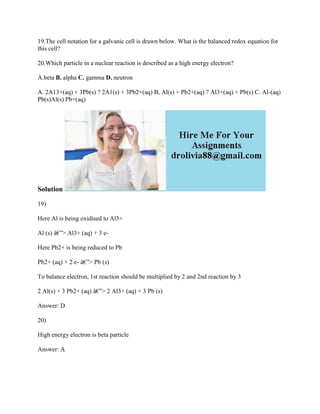Successfully reported this slideshow.

# 19-The cell notation for a galvanic cell is drawn below- What is the b.docx×

# 19-The cell notation for a galvanic cell is drawn below- What is the b.docx

19.The cell notation for a galvanic cell is drawn below. What is the balanced redox equation for this cell?
20.Which particle in a nuclear reaction is described as a high energy electron?
A.beta B. alpha C. gamma D. neutron
A. 2A13+(aq) + 3Pb(s) ? 2A1(s) + 3Pb2+(aq) B, Al(s) + Pb2+(aq) ? Al3+(aq) + Pb(s) C. Al-(aq) Pb(s)Al(s) Pb+(aq)
Solution
19)
Here Al is being oxidised to Al3+
Al (s) â€”> Al3+ (aq) + 3 e-
Here Pb2+ is being reduced to Pb
Pb2+ (aq) + 2 e- â€”> Pb (s)
To balance electron, 1st reaction should be multiplied by 2 and 2nd reaction by 3
2 Al(s) + 3 Pb2+ (aq) â€”> 2 Al3+ (aq) + 3 Pb (s)
20)
High energy electron is beta particle
.

19.The cell notation for a galvanic cell is drawn below. What is the balanced redox equation for this cell?
20.Which particle in a nuclear reaction is described as a high energy electron?
A.beta B. alpha C. gamma D. neutron
A. 2A13+(aq) + 3Pb(s) ? 2A1(s) + 3Pb2+(aq) B, Al(s) + Pb2+(aq) ? Al3+(aq) + Pb(s) C. Al-(aq) Pb(s)Al(s) Pb+(aq)
Solution
19)
Here Al is being oxidised to Al3+
Al (s) â€”> Al3+ (aq) + 3 e-
Here Pb2+ is being reduced to Pb
Pb2+ (aq) + 2 e- â€”> Pb (s)
To balance electron, 1st reaction should be multiplied by 2 and 2nd reaction by 3
2 Al(s) + 3 Pb2+ (aq) â€”> 2 Al3+ (aq) + 3 Pb (s)